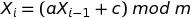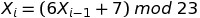## A Linear Congruential Generator (LCG) in R

In my simulation classes, we talk about how to generate random numbers. One of the techniques we talk about is the Linear Congruential Generator (LCG). Starting with a seed, the LCG produces the first number in the sequence, and then uses that value to generate the second one. The second value is used to generate the third, the third to generate the fourth, and so on. The equation looks like this:where a is a multiplier, c is a shift, and m is a modulus. (Modulus just means “find me the remainder when you divide the stuff to the left of the mod operator by the stuff to the right”. Fortunately there is an easy way to do this in R: a mod b is expressed as a %% b.) When you use the LCG to generate a stream of random numbers, they will always be between 0 and (m-1), and the sequence will repeat every m occurrences. When you take the sequence of values x and divide it by the mod m, you get uniformly distributed random numbers between 0 and 1 (but never quite hitting 1). The LCG is a pseudorandom number generator because after a while, the sequence in the stream of numbers will begin to repeat.

I wrote an exam last week with some LCG computations on it, but then I got lazy and didn’t want to do the manual calculations… so I wrote a function in R. Here it is. It returns two different vectors: the collection of numbers between 0 and (m – 1) that the LCG produces, and the collection of uniformly distributed random numbers between 0 and 1 that we get by dividing the first collection by m:

```lcg <- function(a,c,m,run.length,seed) {
x <- rep(0,run.length)
x <- seed
for (i in 1:(run.length-1)) {
x[i+1] <- (a * x[i] + c) %% m
}
U <- x/m # scale all of the x's to
# produce uniformly distributed
# random numbers between [0,1)
return(list(x=x,U=U))
}```

So for example, if your LCG looks like this:Then, after loading in the function above, you could choose a seed (say, 5) and generate a stream of 20 random numbers:

```> z <- lcg(6,7,23,20,5)
> z
\$x
 5 14 22 1 13 16 11 4 8 9 15 5 14 22 1 13 16 11
 4 8

\$U
 0.21739130 0.60869565 0.95652174 0.04347826 0.56521739
 0.69565217 0.47826087 0.17391304 0.34782609 0.39130435
 0.65217391 0.21739130 0.60869565 0.95652174 0.04347826
 0.56521739 0.69565217 0.47826087 0.17391304 0.34782609```

The values contained within z\$x are the numbers that the LCG produces, and the values contained within z\$U are the values of z\$x scaled to fall between 0 and 1. Both z\$x and z\$U are uniformly distributed, although the pattern will become more apparent as you generate more and more random numbers in your stream. Here’s what they look like with 10000 numbers in the stream:

```> z <- lcg(6,7,23,10000,5)
> par(mfrow=c(1,2))
> hist(z\$x,col="red")
> hist(z\$U,col="purple")```But how good is your random number generator? First, you know you want a large (and preferably odd valued mod). That way, the numbers will not repeat so quickly. But there are also considerations dealing with what multiplier and shift you should use. I like checking out the quality of my random number generator by plotting how they move in sequence around a two dimensional grid: the better the random number generator, the more filling of the space you will see.

You can play around with these plots to find a “good” random number generator:

```> z1 <- lcg(6,7,23,2000,5) # "Bad" LCG
> z2 <- lcg(1093,18257,86436,2000,12) # "Good" LCG
> par(mfrow=c(1,2))
> plot( z1\$U[1:(length(z1\$U)-1)], z1\$U[2:length(z1\$U)] , main="Bad")
> plot( z2\$U[1:(length(z2\$U)-1)], z2\$U[2:length(z2\$U)] , main="Good")```Although there are many heuristics you can use to avoid creating a “bad” random number generator, it’s really difficult to design a “good” one. People make careers out of this. I don’t claim to be an expert, I just know how to test to see if a random number generator is suitable for my simulations. (I also really like the handout at http://www.sci.csueastbay.edu/~btrumbo/Stat3401/Hand3401/CongGenIntroB.pdf that explains this graphing approach more, and also talks about how you have to look at patterns in three dimensions as well. When I was searching around for a “good LCG that would give me a nice graph” I found this handout.)